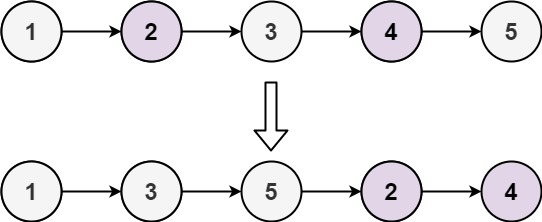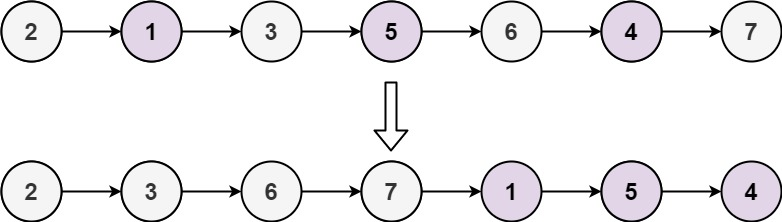## Algorithm

Problem Name: 328. Odd Even Linked List

Given the `head` of a singly linked list, group all the nodes with odd indices together followed by the nodes with even indices, and return the reordered list.

The first node is considered odd, and the second node is even, and so on.

Note that the relative order inside both the even and odd groups should remain as it was in the input.

You must solve the problem in `O(1)` extra space complexity and `O(n)` time complexity.

Example 1:```Input: head = [1,2,3,4,5]
Output: [1,3,5,2,4]
```

Example 2:```Input: head = [2,1,3,5,6,4,7]
Output: [2,3,6,7,1,5,4]
```

Constraints:

• The number of nodes in the linked list is in the range `[0, 104]`.
• `-106 <= Node.val <= 106`

## Code Examples

### #1 Code Example with Java Programming

```Code - Java Programming```

``````
class Solution {
}
ListNode evenIdxNode = evenIdxNodeStart;
while (evenIdxNode != null && evenIdxNode.next != null) {
oddIdxNode.next = evenIdxNode.next;
oddIdxNode = oddIdxNode.next;
evenIdxNode.next = oddIdxNode.next;
evenIdxNode = evenIdxNode.next;
}
oddIdxNode.next = evenIdxNodeStart;
}
}
``````
Copy The Code &

Input

cmd

Output

cmd
[1,3,5,2,4]

### #2 Code Example with Javascript Programming

```Code - Javascript Programming```

``````
if (head === null) return null;
while (even !== null && even.next !== null) {
odd.next = even.next;
odd = odd.next;
even.next = odd.next;
even = even.next;
}
};
``````
Copy The Code &

Input

cmd

Output

cmd
[1,3,5,2,4]

### #3 Code Example with Python Programming

```Code - Python Programming```

``````
class Solution:
"""
:rtype: ListNode
"""
root, i, last, first = head, 1, None, None
if i%2 != 0: last = head
if last: last.next = first
return root
``````
Copy The Code &

Input

cmd

### #4 Code Example with C# Programming

```Code - C# Programming```

``````
namespace LeetCode
{
{
{
if (head == null) return null;
while (even != null && even.next != null)
{
odd.next = even.next;
odd = odd.next;
even.next = odd.next;
even = even.next;
}

}
}
}
``````
Copy The Code &

Input

cmd